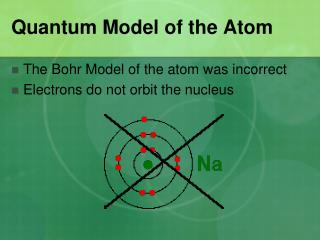DownloadDownload PresentationQuantum Model of the Atom

# Quantum Model of the Atom

Download Presentation## Quantum Model of the Atom

- - - - - - - - - - - - - - - - - - - - - - - - - - - E N D - - - - - - - - - - - - - - - - - - - - - - - - - - -
##### Presentation Transcript

1. Quantum Model of the Atom • The Bohr Model of the atom was incorrect • Electrons do not orbit the nucleus

2. Quantum Model of the Atom • In the modern (quantum) model of the atom, electrons are found in regions of space called orbitals • Orbitals are clouds of probability where electrons are most likely to be found • The shape of these clouds is the result of plotting the solutions to very complicated mathematical equations • We will study 3 types: s, p, and d

3. Quantum Model of the Atom • There are 7 periods on the periodic table • Each period represents the number of energy levels in an atom • Each energy level is represented by “n”

4. Structure of the Quantum Atom • Structure of the electron cloud • Principle energy levels (n) • Obtained from the Periodic Table • Are whole numbers: 1, 2, 3, 4…. • Sublevels • s, p, d, f • Orbitals – a MAX of two electrons are allowed in each orbital for each value of n: • s has one obital • p has three • d has 5 • Spin • Obtained from the Periodic Table

5. Quantum Model of the Atom “s” orbitals • Spherically shaped • There is one “s” orbital for each energy level

6. Quantum Model of the Atom This is the probability diagram of an “s” orbital n = 2 This is a “2 s” orbital n = 1 This is a “1 s” orbital

7. Quantum Model of the Atom “p” orbitals • Dumbbell shaped, with three different orientations in space • Starting with n = 2, there are THREE “p” orbitals, px, py, pz for each energy level

8. Quantum Model of the Atom “d” orbitals • Dumbbell shaped • Starting with n=3, there are 5 “d” orbitals

9. Quantum Model of the Atom How electrons fill their orbitals Pauli Exclusion Principle • Each orbital can hold a maximum of TWO ELECTRONS Aufbau Principle • Electrons fill orbitals that have the LOWEST ENERGY FIRST

10. The Quantum Model of the Atom Filling sequence: 1s<2s<2p<3s<3p<4s<3d Each orbital can hold a maximum of 2 electrons

11. Quantum Model of the Atom Example: Hydrogen • Has one electron • The first energy level to fill is the 1s orbital • The one electron will fill in this orbital • 1s1 1s1 Orbital Number of Electrons in Orbital Energy Level

12. Quantum Model of the Atom Electron Configurations • The arrangement of electrons in an atom • Filling pattern: 1s<2s<2p<3s<3p<4s<3d…….. Or use the following:

13. Quantum Model of the Atom • 1s<2s<2p<3s<3p<4s<3d…….. Filling Pattern

14. Model of the atom with s and p orbitals